# GMAT Math : DSQ: Calculating an angle in a polygon

## Example Questions

2 Next →

### Example Question #151 : Geometry

A nonagon is a nine-sided polygon.

Is Nonagon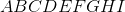regular?

Statement 1: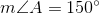Statement 2: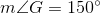Statement 1 ALONE is sufficient to answer the question, but Statement 2 ALONE is NOT sufficient to answer the question.

Statement 2 ALONE is sufficient to answer the question, but Statement 1 ALONE is NOT sufficient to answer the question.

BOTH statements TOGETHER are sufficient to answer the question, but NEITHER statement ALONE is sufficient to answer the question.

BOTH statements TOGETHER are insufficient to answer the question.

EITHER statement ALONE is sufficient to answer the question.

EITHER statement ALONE is sufficient to answer the question.

Explanation:

Each angle of a regular nonagon measures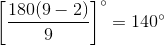Therefore, each of the two statements proves that the nonagon is not regular.

### Example Question #11 : Polygons

What is the measure of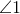?

Statement 1:is an exterior angle of an equilateral triangle.

Statement 2:is an interior angle of a regular hexagon.

BOTH statements TOGETHER are sufficient to answer the question, but NEITHER statement ALONE is sufficient to answer the question.

Statement 1 ALONE is sufficient to answer the question, but Statement 2 ALONE is NOT sufficient to answer the question.

Statement 2 ALONE is sufficient to answer the question, but Statement 1 ALONE is NOT sufficient to answer the question.

EITHER statement ALONE is sufficient to answer the question.

BOTH statements TOGETHER are insufficient to answer the question.

EITHER statement ALONE is sufficient to answer the question.

Explanation:

An exterior angle of an equilateral triangle measures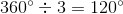. An interior angle of a regular hexagon measures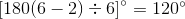. Either statement is sufficient.

### Example Question #151 : Geometry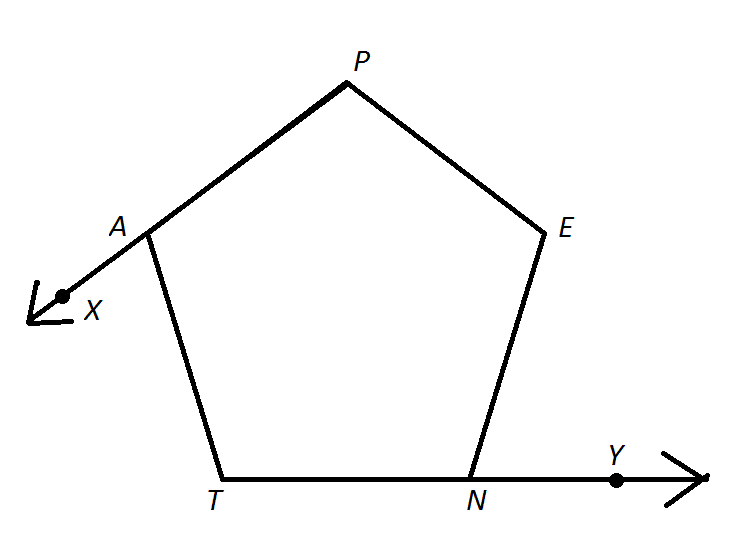Note: Figure NOT drawn to scale.

Refer to the above figure. Is Pentagon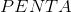regular?

Statement 1: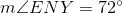Statement 2: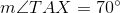Statement 1 ALONE is sufficient to answer the question, but Statement 2 ALONE is NOT sufficient to answer the question.

Statement 2 ALONE is sufficient to answer the question, but Statement 1 ALONE is NOT sufficient to answer the question.

BOTH statements TOGETHER are insufficient to answer the question.

EITHER statement ALONE is sufficient to answer the question.

BOTH statements TOGETHER are sufficient to answer the question, but NEITHER statement ALONE is sufficient to answer the question.

Statement 2 ALONE is sufficient to answer the question, but Statement 1 ALONE is NOT sufficient to answer the question.

Explanation:

If a shape is regular, that means that all of its sides are equal. It also means that all of its interior angles are equal. Finally, if all of the interior angles are equal, then the exterior angles will all be equal to each other as well.

In a regular polygon, we can find the measure of ANY exterior angle by using the formula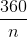whereis equal to the number of sides.

Each exterior angle of a regular (five-sided) pentagon measures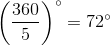Statement 1 alone neither proves nor disproves that the pentagon is regular. We now know that one exterior angle is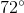, but we do not know if any of the other exterior angles are also.

Statement 2, however, proves that the pentagon is not regular, as it has at least one exterior angle that does not have measure.

2 Next →

### All GMAT Math Resources